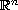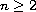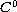Electron. J. Diff. Eqns., Vol. 2008(2008), No. 24, pp. 1-15.

### Growth rate and existence of solutions to Dirichlet problems for prescribed mean curvature equations on unbounded domains Zhiren Jin

Abstract:
We prove growth rate estimates and existence of solutions to Dirichlet problems for prescribed mean curvature equation on unbounded domains inside the complement of a cone or a parabola like region in(). The existence results are proved using a modified Perron's method by which a subsolution is a solution to the minimal surface equation, while the role played by a supersolution is replaced by estimates on the uniformbounds on the liftings of subfunctions on compact sets.

Submitted February 9, 2008. Published February 22, 2008.
Math Subject Classifications: 35J25, 35J60, 35J65.
Key Words: Elliptic boundary-value problem; quasilinear elliptic equation; prescribed mean curvature equation; unbounded domain; Perron's method

Show me the PDF file (281 KB), TEX file, and other files for this article.Zhiren Jin Department of Mathematics and Statistics Wichita State University Wichita, Kansas 67260-0033, USA email: zhiren@math.wichita.edu